Friday , July 10 2020# NCERT 5th Class (CBSE) Mathematics: Order Of Operations

(b) 18 + (10 + 2) ÷ 1/3 of 9

= 18 + (10 + 2) ÷ 1/3 of 9       first do the operation within the brackets

= 18 + 12 ÷ 1/3 of 9 = 18 + 12 ÷ 1/3 × 9       next, perform the ‘of’ operation

= 18 + 12 ÷ 3          then do division

= 18 + 4 = 22          finally, add

18 + (10 + 2) ÷ 1/3 of 9 = 22

(c) 7.2 × 1.1 + ( 4.8 – 1.2 )

= 7.2 × 1.1 + ( 4.8 – 1.2 )         first do the operation within the brackets

=  7.2 × 1.1 + 3.6                      next, multiply

=  7.92 + 3.6 = 11.52               finally, add

7.2 × 1.1 + ( 4.8 – 1.2 ) = 11.52

#### D. Different kinds of brackets can be used in an expression. Each has an order in the way they are placed as well as order in which they have to be simplified and removed.

The following list gives the different kinds of brackets starting from the innermost and going to the outermost.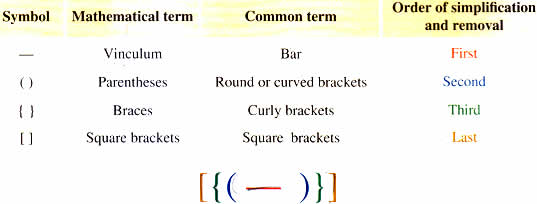Look at these examples: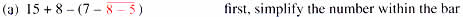= 15 + 8 – ( 7 – 3 )                  next, remove the round brackets

= 15 + 8 – 4                             Then, add

= 23 – 4 = 19                           finally, subtract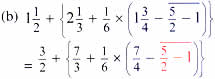Change all mixed numbers to improper fractions and work within the bar

= 3/2 + { 7/3 + 1/6 × ( 7/4 – 3/2) } Remove the bar 5/2 – 1 = 3/2 and work within the round bracket

= 3/2 + { 7/3 + 1/6 × 1/4 } Remove the curved bracket 7/4 – 3/2 = 1/4 and work within the curly bracket

= 3/2 + { 7/3 + 1/24 } Follow BODMAS within curly bracket – multiply 1/6 × 1/4 = 1/24

= 3/2 + 19/8  Remove the curly bracket 7/3 + 1/24 = 57/24 = 19/8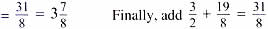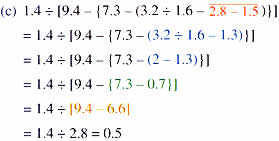## 10th Class CBSE Mathematics 2018-19

10th Class CBSE Mathematics 2018-19 Time: 3 hours M.M.: 80 Class: 10th Subject: Mathematics Date: 07/03/2019 …